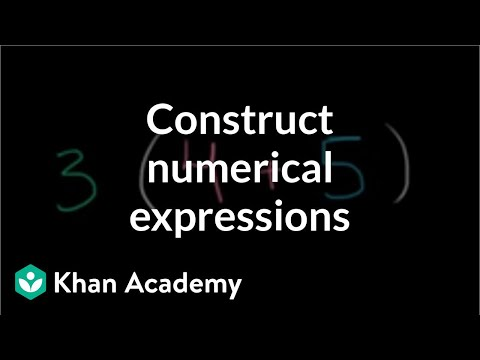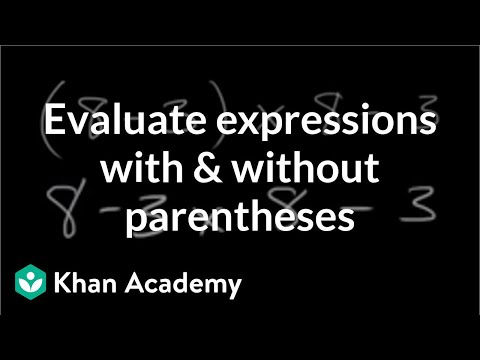# Translating expressions with parentheses (Full video)

Description: A short lesson on interpreting written statements and writing them as mathematical expressions. What I hope to do in this video, is give ourselves some practice interpreting statements and writing them as mathematical expressions, possibly using parentheses. And for any of these statements, if you get so inspired, and I encourage you to get so inspired, pause the video and see if you can write them as mathematical expressions. So we could say, another way to think about divided in half is divided by two, so we could write this as 709 minus 19, and we're going to do that first, so that's why I put the parentheses around it, divided by two, or divided in half.

### Other videos you might be interested in### Constructing numerical expressions (Full video)